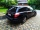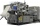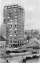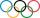# Fraction calculator

The calculator performs basic and advanced operations with fractions, expressions with fractions combined with integers, decimals, and mixed numbers. It also shows detailed step-by-step information about the fraction calculation procedure. Solve problems with two, three, or more fractions and numbers in one expression.

## Result:

### 12/5 - 6/10 = 4/5 = 0.8

Spelled result in words is four fifths.

### How do you solve fractions step by step?

1. Conversion a mixed number 1 2/5 to a improper fraction: 1 2/5 = 1 2/5 = 1 · 5 + 2/5 = 5 + 2/5 = 7/5

To find new numerator:
a) Multiply the whole number 1 by the denominator 5. Whole number 1 equally 1 * 5/5 = 5/5
b) Add the answer from previous step 5 to the numerator 2. New numerator is 5 + 2 = 7
c) Write a previous answer (new numerator 7) over the denominator 5.

One and two fifths is seven fifths
2. Subtract: 7/5 - 6/10 = 7 · 2/5 · 2 - 6/10 = 14/10 - 6/10 = 14 - 6/10 = 8/10 = 2 · 4/2 · 5 = 4/5
For adding, subtracting, and comparing fractions, it is suitable to adjust both fractions to a common (equal, identical) denominator. The common denominator you can calculate as the least common multiple of both denominators - LCM(5, 10) = 10. In practice, it is enough to find the common denominator (not necessarily the lowest) by multiplying the denominators: 5 × 10 = 50. In the next intermediate step, , cancel by a common factor of 2 gives 4/5.
In words - seven fifths minus six tenths = four fifths.

#### Rules for expressions with fractions:

Fractions - use the slash “/” between the numerator and denominator, i.e., for five-hundredths, enter 5/100. If you are using mixed numbers, be sure to leave a single space between the whole and fraction part.
The slash separates the numerator (number above a fraction line) and denominator (number below).

Mixed numerals (mixed fractions or mixed numbers) write as non-zero integer separated by one space and fraction i.e., 1 2/3 (having the same sign). An example of a negative mixed fraction: -5 1/2.
Because slash is both signs for fraction line and division, we recommended use colon (:) as the operator of division fractions i.e., 1/2 : 3.

Decimals (decimal numbers) enter with a decimal point . and they are automatically converted to fractions - i.e. 1.45.

The colon : and slash / is the symbol of division. Can be used to divide mixed numbers 1 2/3 : 4 3/8 or can be used for write complex fractions i.e. 1/2 : 1/3.
An asterisk * or × is the symbol for multiplication.
Plus + is addition, minus sign - is subtraction and ()[] is mathematical parentheses.
The exponentiation/power symbol is ^ - for example: (7/8-4/5)^2 = (7/8-4/5)2

#### Examples:

subtracting fractions: 2/3 - 1/2
multiplying fractions: 7/8 * 3/9
dividing Fractions: 1/2 : 3/4
exponentiation of fraction: 3/5^3
fractional exponents: 16 ^ 1/2
adding fractions and mixed numbers: 8/5 + 6 2/7
dividing integer and fraction: 5 ÷ 1/2
complex fractions: 5/8 : 2 2/3
decimal to fraction: 0.625
Fraction to Decimal: 1/4
Fraction to Percent: 1/8 %
comparing fractions: 1/4 2/3
multiplying a fraction by a whole number: 6 * 3/4
square root of a fraction: sqrt(1/16)
reducing or simplifying the fraction (simplification) - dividing the numerator and denominator of a fraction by the same non-zero number - equivalent fraction: 4/22
expression with brackets: 1/3 * (1/2 - 3 3/8)
compound fraction: 3/4 of 5/7
fractions multiple: 2/3 of 3/5
divide to find the quotient: 3/5 ÷ 2/3

The calculator follows well-known rules for order of operations. The most common mnemonics for remembering this order of operations are:
PEMDAS - Parentheses, Exponents, Multiplication, Division, Addition, Subtraction.
BEDMAS - Brackets, Exponents, Division, Multiplication, Addition, Subtraction
BODMAS - Brackets, Of or Order, Division, Multiplication, Addition, Subtraction.
GEMDAS - Grouping Symbols - brackets (){}, Exponents, Multiplication, Division, Addition, Subtraction.
Be careful, always do multiplication and division before addition and subtraction. Some operators (+ and -) and (* and /) has the same priority and then must evaluate from left to right.

## Fractions in word problems:

• The recipeThe recipe they are following requires 7/8 cups of milk, Tom already put 3/8 cups of milk. How much milk should Lea add to follow the recipe?
• Difference of two fractionsWhat is the difference between 1/2 and 1/6? (Write the answer as a fraction in lowest terms. )
• KingKing had four sons. First inherit 1/2, second 1/4, third 1/5 of property. What part of the property was left to the last of the brothers?
• MichaelMichael had a bar of chocolate. He ate 1/2 of it and gave away 1/3. What fraction had he left?Erika’s career consists of filing, typing and answering phones. She spends 1/6 of her time filing and 5/8 of her time typing. What fraction of her time does she spend answering phone calls?
• Hotel 4A 360 room hotel has 1/3 of its rooms occupied at present . How many rooms are empty?
• Visit to grandfatherShane's family traveled 3/10 of the distance to his grandfather’s house on Saturday. They traveled 4/7 of the remaining distance on Sunday. What fraction of the total distance to his grandfather’s house was traveled on Sunday?Pupils doing research which a winter sport do their classmates most popular. They found that 2/5 of classmates would most like to play hockey, skate prefer 2/9 pupils, 3/10 students prefer skiing and 1/15 classmates don't like any winter sport. What propoAnanya has a bunny. She bought 4 7/8 pounds of carrots. She fed her bunny 1 1/4 pounds of carrots the first week. She fed her bunny 5/6 pounds of carrots the second week. All together, how many pounds of carrots did she feed her bunny? 1. Draw a tape diagAfter Claire has read the first 5/8 of the book, there are 120 pages left to read. What is the total number of pages of the book?Billy ate 1 1/4 pizzas and John ate 1 2/3 pizzas. How much more pizza did John eat than Billy?Kevin is baking brownies and needs peanuts.  The recipe calls for 1 and 2/3 cups of peanuts.  Kevin only has 5/6 of a cup of peanuts.  How many more cups of peanuts does Kevin need to have the correct amount?5 2/5 hours a week  mathematics,  3 3/4 hours a week   Natural sciences, 4 3/8 hours a week  Technology . how many hours does  he spend on social sciences if he spend 17 1/2 hours a week for the four subject?